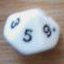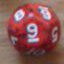Mode  & Freq

## Mode  & Freq

 Thu Nov 28 11:00:14 2019   by   wyrdR Hi Torben,Please consider implementing the following as future functions.mode A :   the most frequent value(s) in collection Afreq {N} A :   returns values from A that appear a number of times matching set N  e.g. {3,5} would return values appearing 3 or 5 timesIt's cool if you can't. I've just been trying to do it with the functions  available already but times tend to balloon out. I thought a built in function might improve things! I might be way off here…Please consider.Thanks in advance, wyrdR Thu Nov 28 11:05:04 2019   by   Torben Hi wyrdR,I doubt it would be much faster to implement them directly in Troll.  These functions do not have nice mathematical properties that can be exploited for optimisation. Thu Nov 28 11:10:06 2019   by   wyrdR Thanks anyway.I thought that might be the case! 😬I guess I'll stick to my own functions. I might submit my best efforts here for your input later.Cheers 🍻 Thu Nov 28 11:31:52 2019   by   wyrdR \ Most Common Dice\ Thanks in advance for any feedbackR:=7d13;'call mode (R) |> 'Rfunction mode(R)=Rx:= max R+1;RC:=different (foreach s in R do Rx*count (R keep s)+s);RCx:=(max RC)/Rx*Rx;foreach s in (RCx < RC) do s mod Rx\ wyrdR 2.19.11.28 Thu Nov 28 11:52:03 2019   by   wyrdR \ Find value by frequencyR:=9d4;'call freq(2,R) <| 'Rfunction freq(F,R)=Rx:= max R+1;Fx:= F*Rx;RC:=different (foreach s in R do Rx*count (R keep s)+s);foreach s in (Fx+Rx)>(Fx <= RC) do s mod Rx\ wyrdR 2.19.11.28 Thu Nov 28 12:33:03 2019   by   wyrdR \ Find the values with Nth highest frequenciesR:=37d20;'call commonest(3,R) <| 'R\ 3 most commonly occurring setsfunction commonest(N,R)=Rx:= max R+1;RC:=different (foreach s in R do Rx*count (R keep s)+s);RCx:=min largest N (  different  (    foreach s in R     do Rx*count (R keep s)  ));foreach s in (RCx < RC) do s mod Rx\ wyrdR 2.19.11.28 Thu Nov 28 23:15:21 2019   by   wyrdR \ Find the values with Nth highest frequenciesR:=37d20;'call commonest(3,R) <| 'R\ 3 most commonly occurring setsfunction commonest(N,R)=Rx:= max R+1;RC:=different (foreach s in R do Rx*count (R keep s)+s);RCx:=min largest N (  different  (    foreach s in R     do Rx*count (R keep s)  ));foreach s in (RCx < RC) do s mod Rx\ wyrdR 2.19.11.28